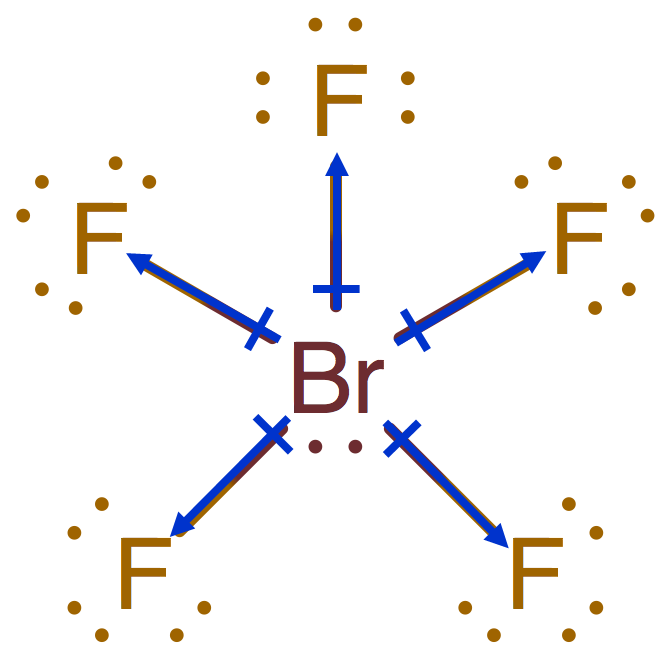# Problem: Bromine pentafluoride, BrF5, is sometimes used as a rocket propellant. Clearly one would like to know quite a bit about the chemical nature of this compound in order to predict how it might behave when loaded into a rocket. Therefore, to answer the questions in this problem, you will need to draw the Lewis structure of bromine pentafluoride. Part AWhat is the electron - domain (charge - cloud) geometry of BrF5? Part BWhat is the molecular geometry of BrF5? Part CIgnoring lone pair effects, what is the smallest bond angle in BrF5? Express your answer as an integer.Part D What is the hybridization about the central atom in BrF5? Express your answer in analogy to the following example: sp^2.Part E Which choice best describes the polarity of BrF5? a) polar bonds / polar molecule b) polar bonds / nonpolar molecule c) nonpolar bonds / polar molecule d) nonpolar bonds / nonpolar molecule

###### FREE Expert Solution

The possible electron pair and molecular geometries are:Step 1: Central atom→ Br (less electronegative than F)

Step 2: Total valence e-

Br      1 × 7 e = 7 e

F       5 × 7 e = 35 e

Total:  42 valence e

Step 3: Lewis Structure99% (124 ratings)###### Problem Details

Bromine pentafluoride, BrF5, is sometimes used as a rocket propellant. Clearly one would like to know quite a bit about the chemical nature of this compound in order to predict how it might behave when loaded into a rocket. Therefore, to answer the questions in this problem, you will need to draw the Lewis structure of bromine pentafluoride.

Part A

What is the electron - domain (charge - cloud) geometry of BrF5

Part B

What is the molecular geometry of BrF5

Part C

Ignoring lone pair effects, what is the smallest bond angle in BrF5? Express your answer as an integer.

Part D

What is the hybridization about the central atom in BrF5? Express your answer in analogy to the following example: sp^2.

Part E

Which choice best describes the polarity of BrF5

a) polar bonds / polar molecule

b) polar bonds / nonpolar molecule

c) nonpolar bonds / polar molecule

d) nonpolar bonds / nonpolar molecule